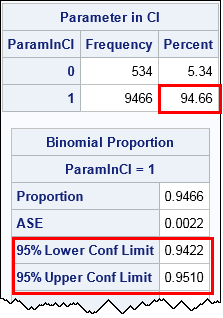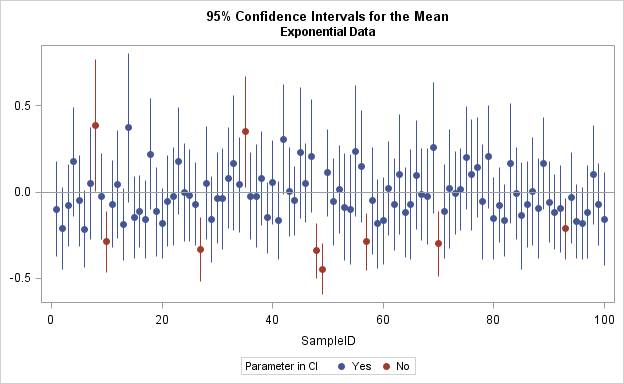The article uses the SAS DATA step and Base SAS procedures to estimate the coverage probability of the confidence interval for the mean of normally distributed data. This discussion is based on Section 5.2 (p. 74–77) of Simulating Data with SAS.

### What is a confidence interval?

Recall that a confidence interval (CI) is an interval estimate that contains the population parameter with a specified probability. Because the CI is an estimate, it is computed from a sample. A confidence interval for a parameter is derived by knowing (or approximating) the sampling distribution of a statistic. For symmetric sampling distributions, the CI often has the form m ± w(α, n), where m is an unbiased estimate of the parameter and w(α, n) is a width that depends on the significance level α, the sample size n, and the standard error of the estimate.

Due to sampling variation, the confidence interval for a particular sample might not contain the parameter. A 95% confidence interval means that if you generate a large number of samples and construct the corresponding confidence intervals, then about 95% of the intervals will contain (or "cover") the parameter.

For example, a well-known formula is the confidence interval of the mean. If the population is normally distributed, then a 95% confidence interval for the population mean, computed from a sample of size n, is
[ xbartc s / sqrt(n),    xbar + tc s / sqrt(n) ]
where

• xbar is the sample mean
• tc = t1-α/2, n-1 is the critical value of the t statistic with significance α and n-1 degrees of freedom
• s / sqrt(n) is the standard error of the mean, where s is the sample standard deviation.

What is a confidence interval? How can you estimate coverage probability? #Statistics #SASTip
Click To Tweet

### Coverage probability

The preceding discussion leads to the simulation method for estimating the coverage probability of a confidence interval. The simulation method has three steps:

1. Simulate many samples of size n from the population.
2. Compute the confidence interval for each sample.
3. Compute the proportion of samples for which the (known) population parameter is contained in the confidence interval. That proportion is an estimate for the empirical coverage probability for the CI.

You might wonder why this is necessary. Isn't the coverage probability always (1-α) = 0.95? No, that is only true when the population is normally distributed (which is never true in practice) or the sample sizes are large enough that you can invoke the Central Limit Theorem. Simulation enables you to estimate the coverage probability for small samples when the population is not normal. You can simulate from skewed or heavy-tailed distributions to see how skewness and kurtosis affect the coverage probability. (See Chapter 16 of Simulating Data with SAS.)

### The simulation method for estimating coverage probability

Let's use simulation to verify that the formula for a CI of the mean is valid when you draw samples from a standard normal population. The following DATA step simulates 10,000 samples of size n=50:

```%let N = 50; /* size of each sample */ %let NumSamples = 10000; /* number of samples */ /* 1. Simulate samples from N(0,1) */ data Normal(keep=SampleID x); call streaminit(123); do SampleID = 1 to &NumSamples; /* simulation loop */ do i = 1 to &N; /* N obs in each sample */ x = rand("Normal"); /* x ~ N(0,1) */ output; end; end; run;```

The second step is to compute the confidence interval for each sample. You can use PROC MEANS to compute the confidence limits. The LCLM= and UCLM= outputs the lower and upper endpoints of the confidence interval to a SAS data set. I also output the sample mean for each sample. Notice that the BY statement is an efficient way to analyze all samples in a simulation study.

```/* 2. Compute statistics for each sample */ proc means data=Normal noprint; by SampleID; var x; output out=OutStats mean=SampleMean lclm=Lower uclm=Upper; run;```

The third step is to count the proportion of samples for which the confidence interval contains the value of the parameter. For this simulation study, the value of the population mean is 0. The following DATA step creates an indicator variable that has the value 1 if 0 is within the confidence interval for a sample, and 0 otherwise. You can then use PROC FREQ to compute the proportion of intervals that contain the mean. This is the empirical coverage probability. If you want to get fancy, you can even use the BINOMIAL option to compute a confidence interval for the proportion.

```/* 3a. How many CIs include parameter? */ data OutStats; set OutStats; label ParamInCI = "Parameter in CI"; ParamInCI = (Lower<0 & Upper>0); /* indicator variable */ run;   /* 3b. Nominal coverage probability is 95%. Estimate true coverage. */ proc freq data=OutStats; tables ParamInCI / nocum binomial(level='1' p=0.95); run;```The output from PROC FREQ tells you that the empirical coverage (based on 10,000 samples) is 94.66%, which is very close to the theoretical value of 95%. The output from the BINOMIAL option estimates that the true coverage is in the interval [0.9422,0.951], which includes 0.95. Thus the simulation supports the assertion that the standard CI of the mean has 95% coverage when a sample is drawn from a normal population.

### Visualizing the simulation study

You can draw a graph that shows how the confidence intervals depend on the random samples. The following graph shows the confidence intervals for 100 samples. The center of each CI is the sample mean.

```proc format; /* display 0/1 as "No"/"Yes" */ value YorN 0="No" 1="Yes"; run;   ods graphics / width=6.5in height=4in; proc sgplot data=OutStats(obs=100); format ParamInCI YorN.; title "95% Confidence Intervals for the Mean"; title2 "Normal Data"; scatter x=SampleID y=SampleMean / group=ParamInCI markerattrs=(symbol=CircleFilled); highlow x=SampleID low=Lower high=Upper / group=ParamInCI legendlabel="95% CI"; refline 0 / axis=y; yaxis display=(nolabel); run;```The reference line shows the mean of the population. Samples for which the population mean is inside the confidence interval are shown in blue. Samples for which the population mean is not inside the confidence interval are shown in red.

You can see how sample variability affects the confidence intervals. In four random samples (shown in red) the values in the sample are so extreme that the confidence interval does not include the population mean. Thus the estimate of the coverage probability is 96/100 = 96% for these 100 samples. This graph shows why the term "coverage probability" is used: it is the probability that one of the vertical lines in the graph will "cover" the population mean.

### The coverage probability for nonnormal data

The previous simulation confirms that the empirical coverage probability of the CI is 95% for normally distributed data. You can use simulation to understand how that probability changes if you sample from nonnormal data. For example, in the DATA step that simulates the samples, replace the call to the RAND function with the following line:

` x = rand("Expo") - 1; /* x + 1 ~ Exp(1) */`

You can then rerun the simulation study. This time the samples are drawn from a (shifted) exponential distribution that has mean 0 and unit variance. The skewness for this distribution is 2 and the excess kurtosis is 6. The result from PROC FREQ is that only about 93.5% of the confidence intervals (using the standard formula) cover the true population mean. Consequently, the formula for the CI, which has 95% coverage for normal data, only has about 93.5% coverage for this exponential data.You can create a graph that visualizes the confidence intervals for the exponential data. Again, only the first 100 samples are shown. In this graph, the CIs for nine samples do not contain the population mean, which implies a 91% empirical coverage.

In summary, you can use simulation to estimate the empirical coverage probability for a confidence interval. In many cases the formula for a CI is based on an assumption about the population distribution, which determines the sampling distribution of the statistic. Simulation enables you to explore how the coverage probability changes when the population does not satisfy the theoretical assumptions.

The post Coverage probability of confidence intervals: A simulation approach appeared first on The DO Loop.